# How To Sum the Largest N Values in Excel

Sometimes we may want to sum the largest N numbers or top N numbers in a range. In this article, we will show you the method of “SUM the Largest N Numbers” by a simple formula which consist of SUMPRODUCT and LARGE functions. LARGE can return largest values based on criteria, SUMPRODUCT can sum up these largest values.

Through a simple instance, we will introduce you the syntax, argument and the usage of SUMPRODUCT and LARGE functions. We will let you know how the formula works step by step clearly. After reading the article, you may know that in which situations we can choose SUMPRODUCT function to sum data.

## EXAMPLE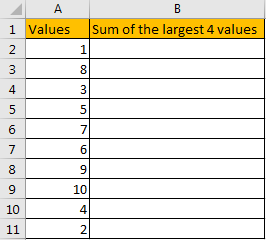In the ‘Values’ column, there are 10 values from ‘1’ to ‘10’ in improper order. Now we want to sum the largest 4 values from this list, how can we get the correct result?

Actually, we can sort these numbers from the largest to the smallest by ‘Sort Z-A’ in excel.

Click ‘Data’ tab, under ‘Sort & Filter’ section click ‘Sort Z to A’ icon.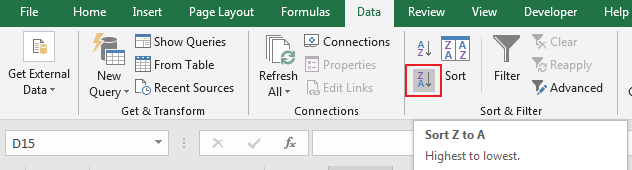Then you can find numbers are ordered properly.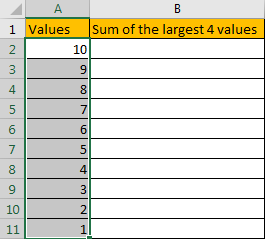Then you can enter SUM function in B2 and select the first four numbers ->cell reference A2:A5 in the list.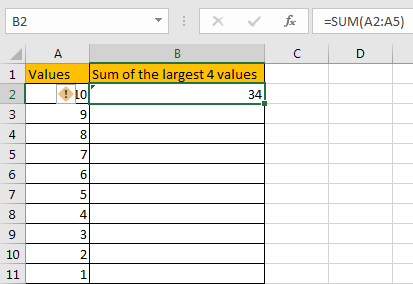Then you can get the sum of the largest four values. It is 34.

In this way, we can get result correctly. But we can also get it conveniently and correctly by just enter a formula. As we want to sum the largest four numbers from range A2:A11, we can find the largest N number or numbers by LARGE function actually, and then use SUMPRODUCT function to sum array directly.

## FORMULA – APPLY SUMPRODUCT FUNCTION

Step 1: In B2, enter the formula

` =SUMPRODUCT(LARGE(A2:A11,{1,2,3,4})).`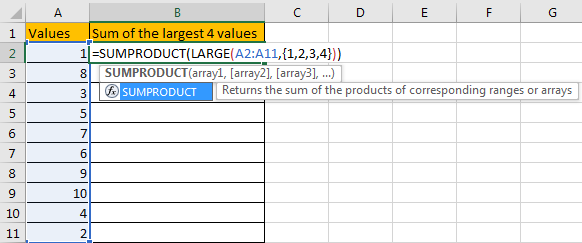Step 2: Press Enter after typing the formula.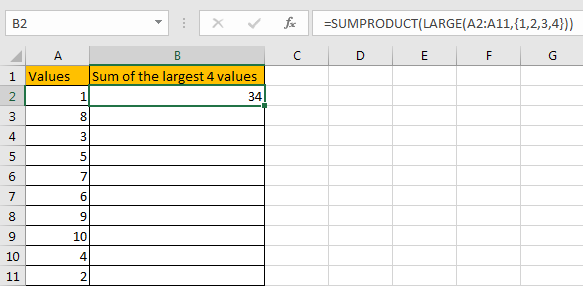We can see that this time we also get correct result 34. The formula works correctly.

## FUNCTION INTRODUCTION

SUMPRODUCT function can be seen as SUM+PRODUCT.

For SUMPRODUCT function, the syntax is:

`=SUMPRODUCT(array1,array2,array3, ...)`

For example, enter =SUMPRODUCT({1,2},{2,3}) in any cell, the we can get value 8, it equals to 1*2+2*3=8. You can also enter =SUMPRODUCT({1,2}*{2,3}), you can get the same result. The argument array can be a real array {1,2,3} for example or a range reference like A2:A7.

If there is only array in the formula, SUMPRODUCT will sum the numbers in the array.

SUMPRODUCT function allows entering texts, logical operators and expressions like (product=cap), texts should be enclosed into ().

LARGE function can return the Nth largest value in a range.

For LARGE function, the syntax is:

`=LARGE(array,k)`

If k is a number, it returns the Kth largest value in the range; if k is an array like {1,2,3}, it returns the largest three values in the range. If k is an array, but the numbers are in inconsecutive sequence for example k={1,2,4}, then LARGE function will return the 1st, 2nd, 4th largest values in the range.

## ARGUMENTS EXPLAINATION

In this formula =SUMPRODUCT(LARGE(A2:A11,{1,2,3,4})), we applied two functions.

For LARGE function, range is A2:A11, k is an array {1,2,3,4}.

Select range A2:A11 in formula bar, press F9 to convert cell reference to real values, numbers in ‘Values’ column are expanded in an array.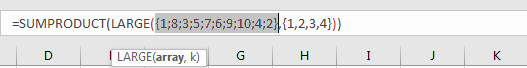For SUMPRODUCT function, there is only one array, it is the result of formula LARGE({1;8;3;5;7;6;9;10;4;2},{1,2,3,4}).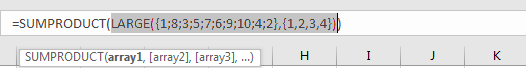## HOW THIS FORMULA WORKS

After explaining argument in the formula, the formula is converted to =SUMPRODUCT(LARGE({1;8;3;5;7;6;9;10;4;2},{1,2,3,4})) in the formula bar. Now we will show you how the formula works with the two functions.

For LARGE({1;8;3;5;7;6;9;10;4;2},{1,2,3,4}), refer to k value {1,2,3,4}, we find out the largest 4 values in the array {1;8;3;5;7;6;9;10;4;2}.

Select LARGE({1;8;3;5;7;6;9;10;4;2},{1,2,3,4}) in the formula bar, press F9. We can get the result {10,9,8,7}. Numbers are ordered from the largest to the smallest.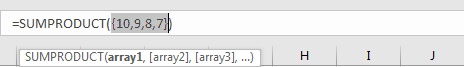Obviously, the final result is 10+9+8+7=34. Select =SUMPRODUCT({10,9,8,7}) in the formula bar, press F9, 34 is displayed in formula bar.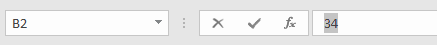1. In this instance, there are only 10 numbers in the range, and we only want to sum the largest 4 numbers. If there are a large amount of numbers, and sum the largest 100 or more numbers in the range, do we need to enter {1,2,3,4,…,100} in LARGE function? Obviously, it is very complex and troublesome. So, we can solve this problem by the help of ROW and INDIRECT

For example, if we want to sum the largest 9 numbers in the same range, we can enter the follow formula in Cell B2:

`=SUMPRODUCT(LARGE(A2:A11,ROW(INDIRECT("1:9"))))`

Then we can get correct value 54.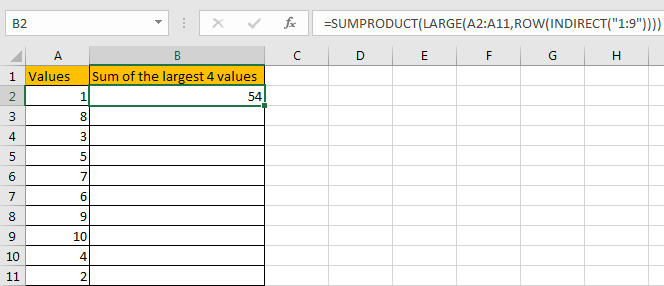In this case, INDIRECT function can return a valid reference based on entered texts. With the help of ROW, ROW(INDIRECT(“1:9”)) can convert “1:9” to {1,2,3,4,5,6,7,8,9}.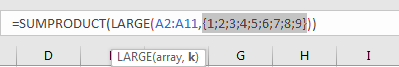2. If N number is saved in another cell, we can concentrate N inside INDIRECT function. Enter the follow formula in Cell d2 this time:

`=SUMPRODUCT(LARGE(A2:A11,ROW(INDIRECT("1:"&D1))))`

See example below: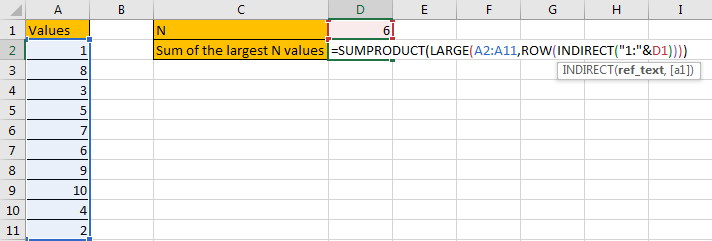In this case, when changing the number in D1, result in D2 will be automatically updated. We don’t need to adjust the formula or cell reference. See example below.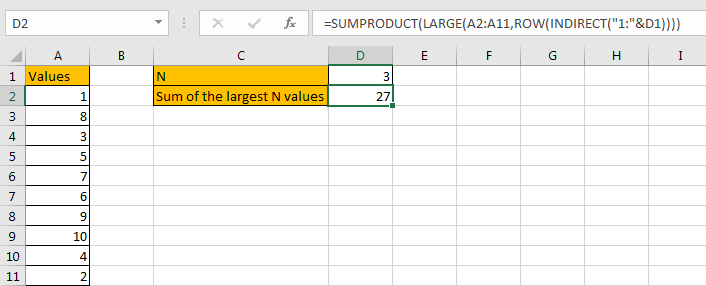3. In this case we can also use SUM function to replace SUMPRODUCT function. But SUMPRODUCT function can handle arrays directly without entering Ctrl + Shift + Enter, so we often apply SUMPRODUCT function in similar situations.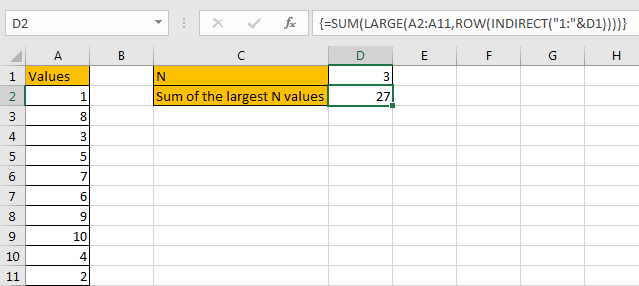SUMMARY

1. SUMPRODUCT function can handle multiple arrays, and it sum up the products.

2. SUMPRODUCT function allows user defined range, wildcards, logical operators, expressions.

3. LARGE function can return the Nth or first Nth largest value in a range.

4. ROW(INDIRECT(“1:N”)) can return a set of numeric numbers in an array.

5. If N is in another cell, use “&” to concentrate them inside INDIRECT, for example ROW(INDIRECT(“1:”&B2)).

### Related Functions

• Excel SUMPRODUCT function
The Excel SUMPRODUCT function multiplies corresponding components in the given one or more arrays or ranges, and returns the sum of those products. The syntax of the SUMPRODUCT function is as below:= SUMPRODUCT (array1,[array2],…)…
• Excel ROW function
The Excel ROW function returns the row number of a cell reference.The ROW function is a build-in function in Microsoft Excel and it is categorized as a Lookup and Reference Function.The syntax of the ROW function is as below:= ROW ([reference])….
• Excel LARGE function
The Excel LARGE function returns the largest numeric value from the numbers that you provided. Or returns the largest value in the array. The syntax of the LARGE function is as below:= LARGE (array,nth)…
• Excel INDIRECT function
The Excel INDIRECT function returns the cell reference based on a text string, such as: type the text string “A2” in B1 cell, it just a text string, so you can use INDIRECT function to convert text string as cell reference….
• Excel SUM function
The Excel SUM function will adds all numbers in a range of cells and returns the sum of these values. You can add individual values, cell references or ranges in excel.The syntax of the SUM function is as below:= SUM(number1,[number2],…)